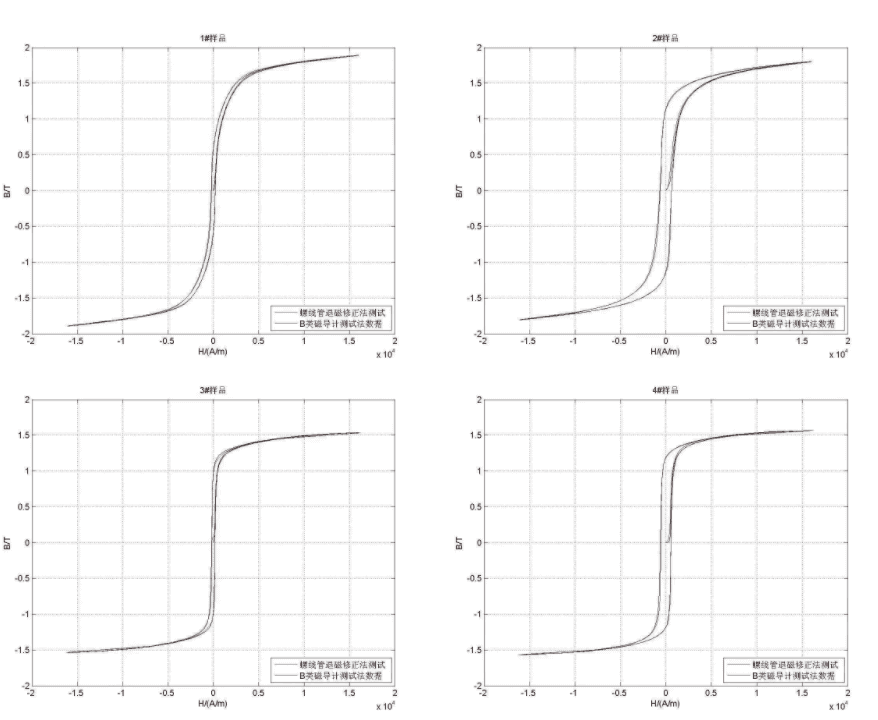#### products categoriesDownload file and products catalogue for equipment.

• Tel : 18030236818
• Fax : +86-592 5237901
• Email : dexing@china-dexing.com

# Measurement of soft magnetic materials: description of the ability to test AC/DC characteristics of magnetic materials1、Measurement of direct current magnetic properties of soft magnetic materials: reference standard：GB/T13012、IEC60404-4

For coercive force is less than 1 ka/m soft magnetic materials materials, mainly include: electrical pure iron, electrician silicon steel, permalloy, amorphous soft magnetic ferrite materials, such as testing usually adopt sealed sample measurement, the test method is: on the closed circuit samples (such as a circle) primary N1 and N2 secondary winding, through to the primary winding is measured, and press: H = N1 * I/Le, to calculate H. Where N1 is the number of turns of the primary excitation winding, I is the excitation current, and Le is the magnetic circuit length of the test sample. The magnetic flux ratio is obtained by integrating the induced voltage of the secondary pole. The magnetic flux is divided by the effective cross-sectional area and the number of induced turns to obtain the magnetic induction intensity, and the value B is obtained. According to the test of B, H value, against the magnetization curve and hysteresis loop, and refer to the definition, determine the corresponding curve on the initial permeability of UI, maximum permeability um, remanence Br, coercive force Hc and saturated magnetic induction intensity, Bs excitation magnetic field intensity of Hs and so on, the magnetic properties of soft magnetic material dc is the most important basic parameters of soft magnetic materials. At present, German Magnet Physik C-300 and 750 are the representative of the test equipment, and a small number of Japanese Metron SK1100 products were also adopted in the early stage. These products are more expensive than domestic equipment, and the magnetization curve and hysteresis loop are measured by scanning method. For soft magnetic measurement with HC less than 1A/m, the test deviation is large. Since the 1990 s, the domestic equipment solves the impact simulation method is used to test the magnetization curve of material, so the initial permeability test UI and maximum permeability um, both in accuracy and repeatability is far superior to similar foreign products, in 2016, xiamen surplus dexing magnetoelectricity technology co., LTD. Has realized the impact simulation method is used to test the hysteresis loop of soft magnetic materials, equipment with foreign counterparts in the process of the test will be on, repetitive appeared a certain advantage.

1) Closed circuit sample measurement: according to the standard, it is suitable for HS below 10kA/m. At present, the user requirements for the powder core sample should be at least 40kA/m, which can be solved when the magnetic ring is large enough to meet the winding of the excitation coil;
2) Rod or strip sample measurement: according to the standard suitable for HS up to 200kA/m. This method should only be suitable for the measurement of hysteresis loops of soft magnetic materials whose HC is greater than 100A/m.
3) It has long been the only method for open sample testing of soft magnetic materials to use A or B type permeability meter to measure open sample. It is difficult to determine the source of test error when there is A dispute between different equipment in the test, so it can only be solved through negotiation.

*** Commonly used AC magnetic characteristic parameter description:

1), than the total loss: Pc Σ Vi * I * 2 = V cosine theta, voltage and current can be obtained by the Angle theta;
2), loss Angle: δ=90 ° -θ;
3), magnetic induction intensity: Bm=V2/ (4.44× F ×N2× AE) (V2 induced voltage effective value, N2 secondary turns, AE effective cross-sectional area);
4). Exciting field intensity: Hm = N1×I1/Le (I1 takes the peak current and Le is the effective magnetic circuit length);
5), amplitude permeability: A = Bm/Hm;
6), elastic permeability: μ '= cosδ× uA, represents the proportion coefficient in the magnetic induction component in the same phase with the magnetic field intensity;
7), viscosity permeability: "= sinδ× uA, representing the proportion coefficient of the magnetic induction component and the magnetic field strength behind π/2 part;
8), quality factor: Q value = μ '/ μ ", material U "requirements as low as possible;
9) Inductance permeability: Ul = uA/COS δ, equivalent parallel inductance permeability;
10), resistive permeability: UR =Q×uL, equivalent parallel resistive permeability;
11), inductance coefficient: Al =cosδ× UA ×4π×10-4× AE/LE;
12) Total inductance: L=N×N× Al.